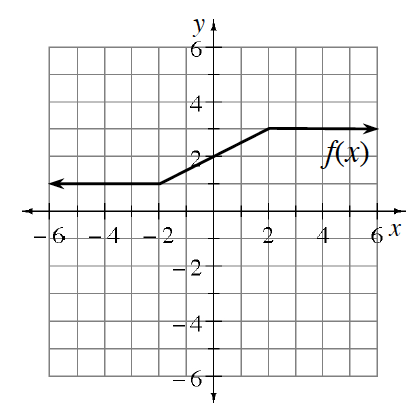### Home > INT1 > Chapter Ch11 > Lesson 11.1.2 > Problem11-22

11-22.

Given the graph of $f(x)$ at right, graph each transformation listed below.

1. $f(x)−3$

Subtract 3 from each y-value on the graph. How will this shift the graph?

2. $f(x)+2$

Refer to part (a).